# Exercise 14.3 Statistics NCERT Solutions Class 9

## Chapter 14 Ex.14.3 Question 1

A survey conducted by an organization for the cause of illness and death among the women between the ages $$15 - 44$$ (in years) worldwide, found the following figures (in $$\%$$):

 S. No. Causes Female fatality rate (%) $$1.$$ Reproductive health conditions $$31.8$$ $$2.$$ Neuropsychiatric conditions $$25.4$$ $$3.$$ Injuries $$12.4$$ $$4.$$ Cardiovascular conditions $$4.3$$ $$5.$$ Respiratory conditions $$4.1$$ $$6.$$ Other causes $$22.0$$
1. Represent the information given above graphically.
2. Which condition is the major cause of women’s ill health and death worldwide?
3. Try to find out, with the help of your teacher, any two factors which play a major role in the cause in (ii) above being the major cause.

### Solution

What is known?

Female fatality rate($$\%$$)

What is Unknown?

1. Represent the given information graphically
2. Major cause of women’s ill health and death worldwide.
3. Factors that play a major role in the major cause of women’s illness and death.

Reasoning:

The above data can be represented graphically using a bar graph as below

1. We will represent the causes on the $$x$$-axis, maintaining equal widths for all bars and with equal gaps in between. One cause will be represented by one unit.
2. We will represent the fatality rate on the y-axis with a scale of unit as $$5\%$$ as the max value.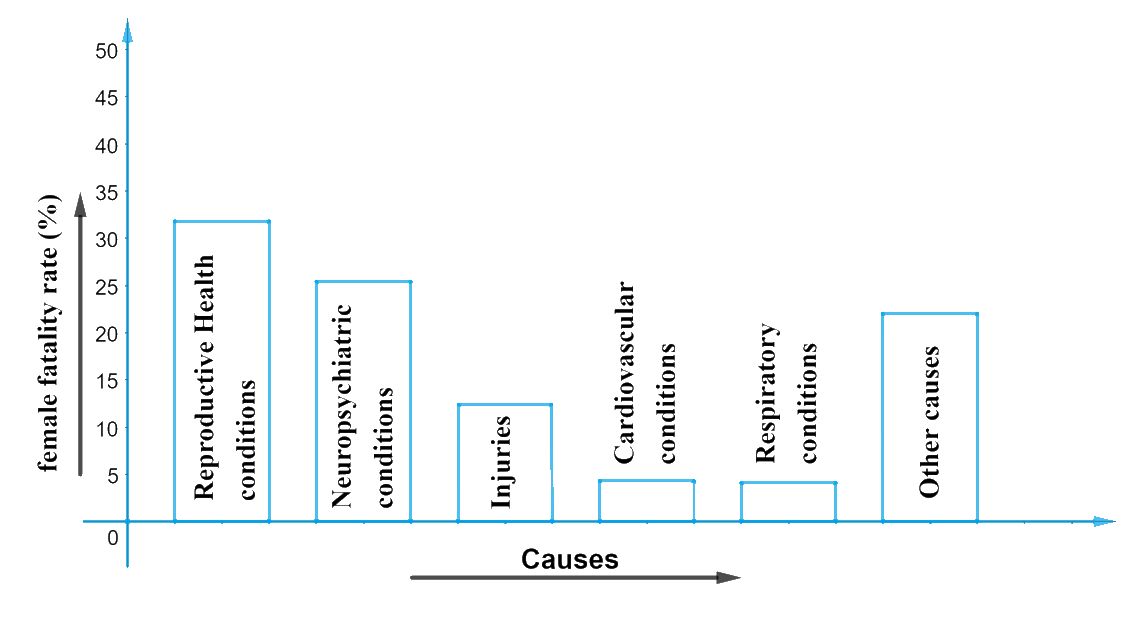It can be visualized from the graph that

1. Fatality rate due to reproductive health condition is more than double that of fatality rate owing to ‘injuries. Reproductive health condition can be considered as the major cause of women’s ill health worldwide.
2. The factors contributing to (i) can be as follows:
(a) Lack of awareness / knowledge about reproductive health conditions.
(b) Lack of emergency medical facilities.
(c) Knowledge about treatment.
(d) Affordability of treatment cost.

## Chapter 14 Ex.14.3 Question 2

The following data on the number of girls (to the nearest ten) per thousand boys in different sections of Indian society is given below.

 Section Number of girls per thousand boys Scheduled Caste (SC) $$940$$ Scheduled Tribe (ST) $$970$$ Non SC/ST $$920$$ Backward districts $$950$$ Non-backward districts $$920$$ Rural $$930$$ Urban $$910$$

(i) Represent the information above by a bar graph.

(ii) In the classroom discuss what conclusions can be arrived at from the graph.

### Solution

What is known?

The number of girls (to the nearest ten) per thousand boys in different sections of Indian society is given

Unknown:

1. Graphical representation of the given information.
2. Conclusions that can be arrived from the graph.

Reasoning:

The above data can be represented graphically using a bar graph as below:

1. Represent the ‘section’ on the $$x$$-axis and ‘number of girls per thousand boys on the $$y$$-axis.
2. We will select a scale of $$1\,\rm unit = 100$$ girls for $$y$$-axis as the max value did not exceed low.
3. We will maintain equal width for all the bars in $$x$$-axis and also maintain equal gaps in between the bars.

Steps: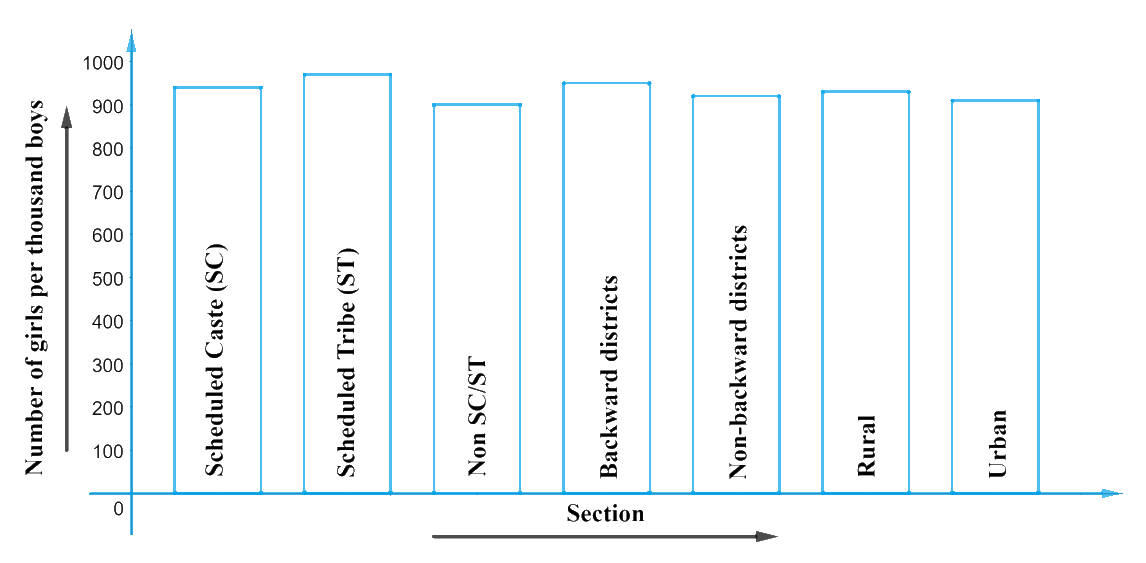It can be observed from the graph that:

1. The number of girls per thousand boys is highest for $$ST$$ category.
2. The number of girls per thousand boys is lowest for urban category.
3. The ‘number of given / $$1000$$’ boys are higher in rural area than in urban.
4. The ‘number of given / $$1000$$’ boys are also higher in backward districts them in non-backward districts.

Point to note:

1. The variations in values occur mostly in the range of $$900-970.$$
2. The values are same until $$900.$$
3. Hence to make the variations more visible and distinct and for a better study of the graph, we can keep the starting value on the $$y$$-axis as ‘$$900$$’ with a scale of $$1\,\rm unit$$ as ‘$$10$$’ and re construct the graph as shown below: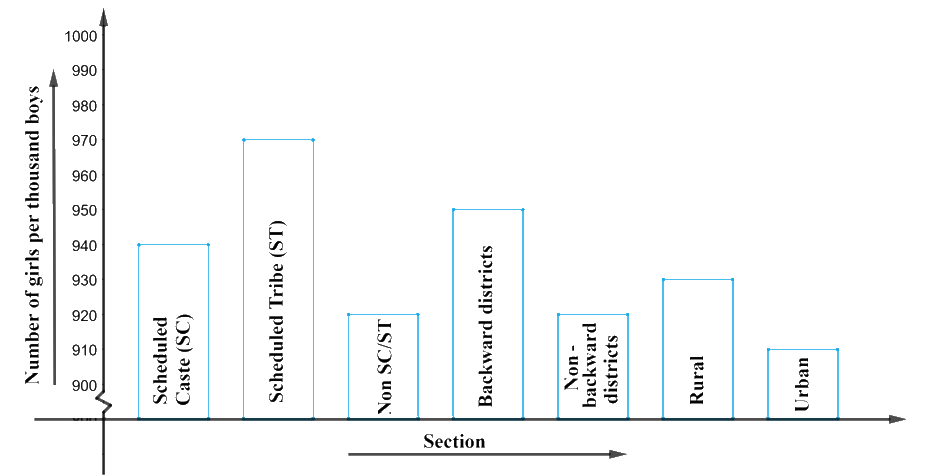Number of girls / thousands of boys

As you can see, the above graph shows a clear picture of the data and helps us to arrive at conclusions easily.

## Chapter 14 Ex.14.3 Question 3

Given below are the seats won by different political parties in the polling outcome of a state assembly elections:

 Political Party $$A$$ $$B$$ $$C$$ $$D$$ $$E$$ $$F$$ Seats Won $$75$$ $$55$$ $$37$$ $$29$$ $$10$$ $$37$$
1. Draw a bar graph to represent the polling results.
2. Which political party won the maximum number of seats?

### Solution

What is known?

The seats won by different political parties in the polling outcome of a state assembly elections.

What is unknown?

1. Bar graph to represent the polling results.
2. The political party which won the maximum number of seats.

Reasoning:

The given data can be represented through a bar graph by:

1. Taking ‘Political Party’ on $$x$$-axis and seats won on $$y$$-axis with a scale of $$1\,\rm unit = 10$$ seats on $$y$$-axis.
2. Maintaining equal width for all the bars in $$x$$-axis with equal gaps in between them.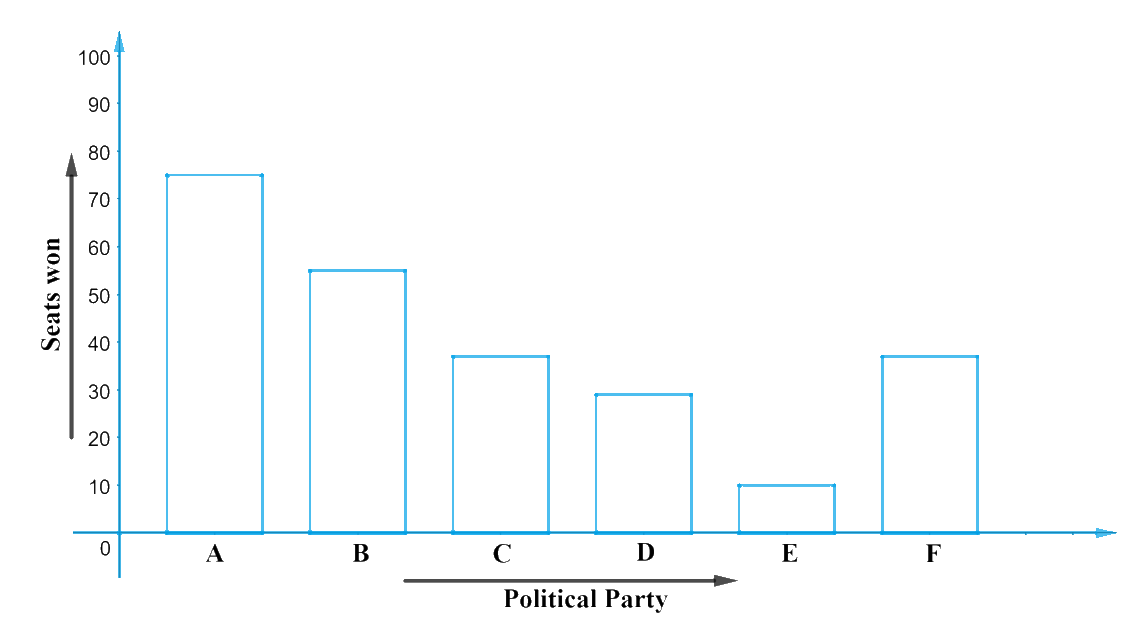From the graph, it can be observed that the political party ‘$$A$$’ won the maximum number of results.

## Chapter 14 Ex.14.3 Question 4

The length of $$40$$ leaves of a plant are measured correct to one millimetre, and the obtained data is represented in the following table:

 Length (in mm) Number of leaves 118– 126 3 127– 135 5 136– 144 9 145– 153 12 154– 162 5 163– 171 4 172- 180 2
1. Draw a histogram to represent the given data. [Hint: First make the class intervals continuous]
2. Is there any other suitable graphical representation for the same data?
3. Is it correct to conclude that the maximum number of leaves are 153 mm long? Why?

### Solution

Difficulty Level: Medium Reasoning:

1. From the given data, it can be observed that the length of leaves are represented in discontinuous class intervals, with a difference of 1 unit in between them.
2. To make the class intervals continuous, we can add 0.5

What is known/given?

The length of 40 leaves of a plant are measured correct to one millimetre, and the obtained data is represented in the table.

What is unknown?

1. A histogram to represent the given data
2. Class interval type
3. Another way of graphical representation of the same data.
4. As certaining the conclusion that the maximum number of leaves are 153 mm long.

Solution:

Find the difference between upper limit of a class and the lower limit of its succeeding class. We then add half of this difference to each of the upper limits and subtract the same from each of the lower limit since the difference is 1 (127 – 126 = 1) half of 1 is 0.5. Now the frequency distribution table will look like as below.

 Length (in mm) Number of Leaves 117.5 – 126.5 3 126.5 – 135.5 5 135.5 – 144.5 9 144.5 – 153.5 12 153.5 – 162.5 5 162.5 – 171.5 4 171.5 – 180.5 2

The above data can be represented graphically through a histogram as below:

1. Represent “Length of leaves (in mm) on x-axis and ‘number of leaves’ in y-axis.
2. And with a scale of ‘1 unit = 2 leaves’, since the lower-class value is 2 and the highest is 12.
3. Also, since the first-class interval is starting from 117.5 and not zero, we show it on the graph by making a kink on a break on the x-axis.
4. We will make now draw rectangular bars of width equal in sizes and lengths according to the frequencies of class intervals. For eg, the rectangular bar for the class interval 117.5 – 126.5 will be of width 1cm and length 1.5 cm.

<Insert image (14.3-5)> It can be observed from the above graph that the other suitable graphical representation of the same data would be a frequency polygon as below: Join the mid points of the upper sides of all the rectangular bars through line segments (as shown in red dotted lines) Let them B, C, D, E, F, G, H. To complete it look like a polygon figure, assume that there is a class interval with ‘0’ frequency, before ‘117.5 – 126.5’, and one after ‘171.5 – 180.5’. Now mark their imaginary mid points as ‘a’ and ‘I’ respectively. Now, “ABCDEFGHI” is the frequency polygon that can be constructed for the given data. (Refer the red dotted line segment) The maximum number of leaves have their length lie between 144.5 mm and 153.5 mm. Hence, we cannot say all leaves have their lengths as 153 mm.

## Chapter 14 Ex.14.3 Question 5

The following table gives the life times of $$400$$ neon lamps:

 Life time (in hours) Number of lamps $$300 - 400$$ $$14$$ $$400 - 500$$ $$56$$ $$500 - 600$$ $$60$$ $$600 - 700$$ $$86$$ $$700 - 800$$ $$74$$ $$800 - 900$$ $$62$$ $$900 - 1000$$ $$48$$

(i) Represent the given information with the help of a histogram.

(ii) How many lamps have a life time of more than $$700$$ hours?

### Solution

What is known?

Life times of $$400$$ neon lamps.

What is Unknown?

(i) A histogram representation for the given data.

(ii) Number of lamps that have a lifetime of more than $$700$$ hours.

Reasoning:

The given data can be represented with the help of a histogram as above:

1. Represent the ‘lifetime (in hours) in $$x$$-axis.
2. Represent the ‘number of lamps’ in $$y$$-axis.
3. Class intervals are continuous.
4. Take “$$1\,\rm unit = 10\,\rm lamps$$” on $$y$$-axis as the lowest value of frequency is $$14$$ and highest $$86.$$
5. Also since the first interval is starting from $$300$$ and not ‘$$0$$’, we show it by marking a ‘kink’ or a break on the $$x$$-axis.

Steps: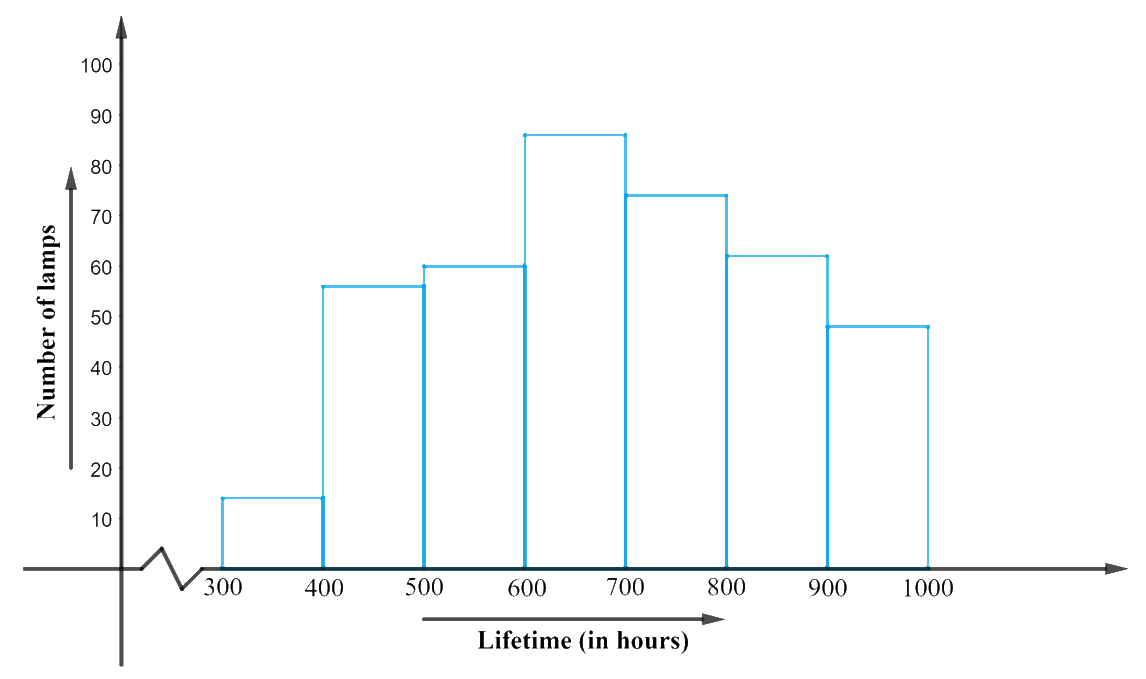From the above graph, it can be concluded that: The number of neon lamps having their lifetime more than $$700$$ is under class intervals “$$700 – 800, 800 – 900, 900 – 1000$$”.

Hence, their corresponding frequencies when added up will be $$(72 + 62 + 48) 184$$ lamps.

## Chapter 14 Ex.14.3 Question 6

The following table gives the distribution of students of two sections according to the marks obtained by them:

 Section A Section B Marks Frequency Marks Frequency $$0-10$$ $$3$$ $$0-10$$ $$5$$ $$10-20$$ $$9$$ $$10-20$$ $$19$$ $$20-30$$ $$17$$ $$20-30$$ $$15$$ $$30-40$$ $$12$$ $$30-40$$ $$10$$ $$40-50$$ $$9$$ $$40-50$$ $$1$$

Represent the marks of the students of both the sections on the same graph by two frequency polygons. From the two polygons compare the performance of the two sections.

### Solution

What is known?

The distribution of students of two sections according to the marks obtained by them.

What is Unknown?

(i) A frequency polygon to represent the marks of the students of both the sections.

(ii) Comparisons of performance of those two sections.

Reasoning:

Frequency polygons can also be drawn independently without drawing histograms. For this requires the midpoints of the class-intervals used in the data. The mid-points are called class-marks.

\text{Class}\,\text{Marks}=\frac{\left( \begin{align}& \text{Upper}\,\text{Limit+} \\ & \text{Lower}\,\text{Limit} \\ \end{align} \right)}{2}

Steps:

Now, the data table with inclusion of class marks:

 Section A Section B Marks Class Mark Frequency Marks Class Mark Frequency $$0-10$$ $$5$$ $$3$$ $$0-10$$ $$5$$ $$5$$ $$10-20$$ $$15$$ $$9$$ $$10-20$$ $$15$$ $$19$$ $$20-30$$ $$25$$ $$17$$ $$20-30$$ $$25$$ $$15$$ $$30-40$$ $$35$$ $$12$$ $$30-40$$ $$35$$ $$10$$ $$40-50$$ $$45$$ $$9$$ $$40-50$$ $$45$$ $$1$$

The frequency polygon for the above data can be constructed by:

• Taking class marks on $$x$$-axis.
• Taking ‘frequency’ on $$y$$-axis with an appropriate scale of $$1\,\rm unit = 2$$ students as the lowest frequency value observed is $$1$$ and the highest frequency value is $$19.$$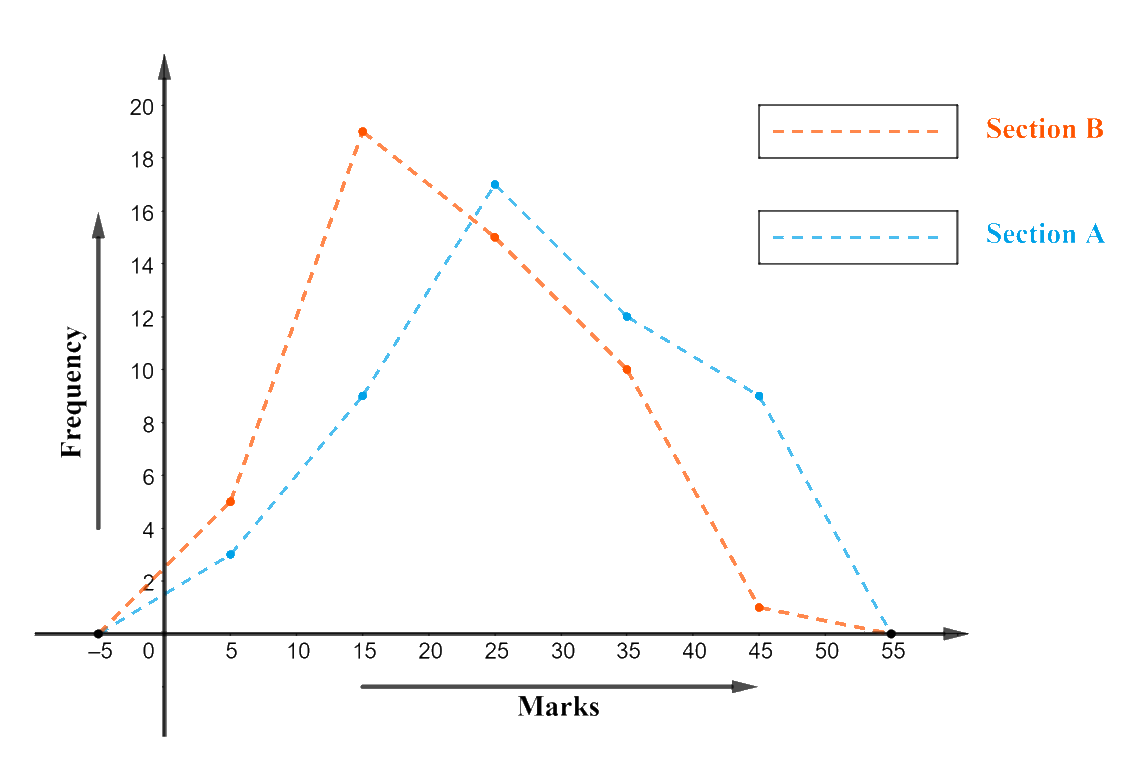It can be observed that the performance of students of Section ‘$$A$$’ is better than the students of Section '$$B$$' as section ‘$$A$$’ shows more students securing marks between ‘$$40 – 50$$’ class and ‘$$30 – 40$$’ class.

## Chapter 14 Ex.14.3 Question 7

The runs scored by two teams $$A$$ and $$B$$ on the first $$60$$ balls in a cricket match are given below:

 Number of balls Team A Team B $$1 – 6$$ $$2$$ $$5$$ $$7 – 12$$ $$1$$ $$6$$ $$13 – 18$$ $$8$$ $$2$$ $$19 – 24$$ $$9$$ $$10$$ $$25 – 30$$ $$4$$ $$5$$ $$31 – 36$$ $$5$$ $$6$$ $$37 – 42$$ $$6$$ $$3$$ $$43 – 48$$ $$10$$ $$4$$ $$49 – 54$$ $$6$$ $$8$$ $$55 – 60$$ $$2$$ $$10$$

Represent the data of both the teams on the same graph by frequency polygons.

[Hint: First make the class intervals continuous.]

### Solution

What is known?

The runs scored by two teams $$A$$ and $$B$$ on the first $$60$$ balls in a cricket match are given.

What is Unknown?

A frequency polygon to represent the data of both the teams.

Reasoning:

1. It can be observed from the given data that the class intervals of the given data are not continuous. There is a gap of ‘$$I$$’ unit between them. So, to make the class intervals continuous, $$0.5$$ has to be added to every upper class limit and $$0.5$$ has to be subtracted from the lower class limit.
2. Class mark should also be found as below:

\text{Class}\,\text{Marks}=\frac{\left( \begin{align} & \text{Upper}\,\text{Limit+} \\ & \text{Lower}\,\text{Limit} \\ \end{align} \right)}{2}

Steps:

The data table with continuous interval and with class mark is as below:

 Number of balls Class Mark Team A Team B $$0.5 \,– 6.5$$ $$3.5$$ $$2$$ $$5$$ $$6.5 \,– 12.5$$ $$9.5$$ $$1$$ $$6$$ $$12.5\, – 18.5$$ $$15.5$$ $$8$$ $$2$$ $$18.5\, – 24.5$$ $$21.5$$ $$9$$ $$10$$ $$24.5 \,– 30.5$$ $$27.5$$ $$4$$ $$5$$ $$30.5 \,– 36.5$$ $$33.5$$ $$5$$ $$6$$ $$36.5 \,– 42.5$$ $$39.5$$ $$6$$ $$3$$ $$42.5 \,– 48.5$$ $$45.5$$ $$10$$ $$4$$ $$48.5\,– 54.5$$ $$51.5$$ $$6$$ $$8$$ $$54.5 \,– 60.5$$ $$57.5$$ $$2$$ $$10$$

The frequency polygon for the above data can be constructed by

(i) Number of balls on $$x$$-axis

(ii) Runs scored on $$y$$-axis with an approximate scale of “$$1\,\rm unit = 1\,\rm run$$” as the lowest run was at $$1$$ and the highest was at $$10.$$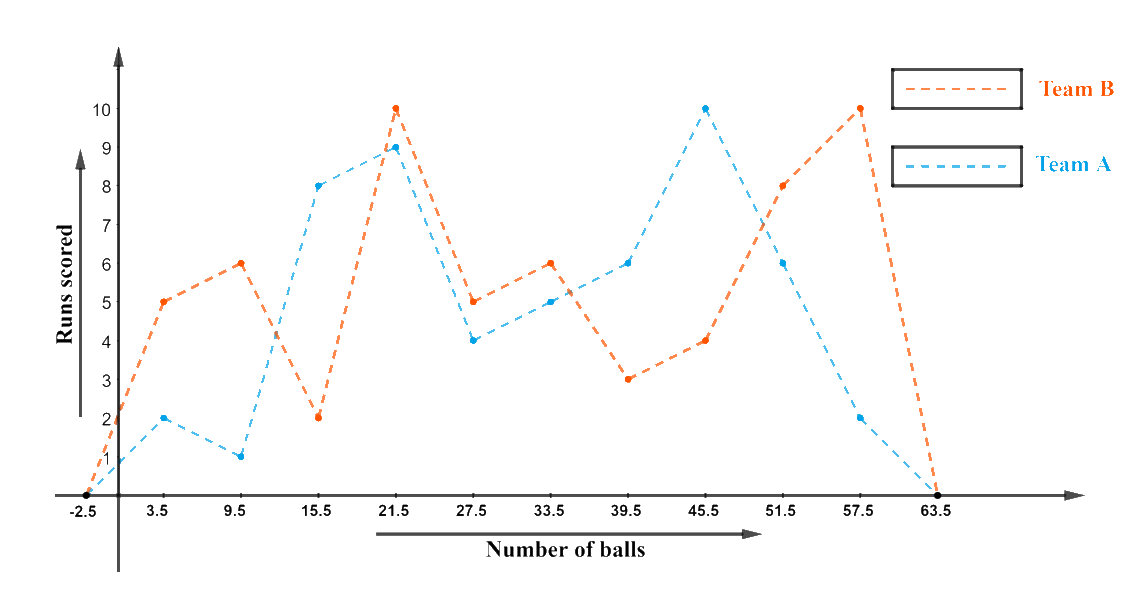## Chapter 14 Ex.14.3 Question 8

A random survey of the number of children of various age groups playing in a park was found as follows:

 Age (in years) Number of children $$1 - 2$$ $$5$$ $$2 – 3$$ $$3$$ $$3 – 5$$ $$6$$ $$5 – 7$$ $$12$$ $$7 – 10$$ $$9$$ $$10 – 15$$ $$10$$ $$15 - 17$$ $$4$$

Draw a histogram to represent the data above.

### Solution

What is known?

A random survey of the number of children of various age groups playing in a park.

What is Unknown?

A Histogram to represent the data.

Reasoning:

(i) From the given data, we can observe that the class intervals have varying width. This will make the rectangular bars to have varying widths and will give us a misleading picture of the data.

(ii) The areas of the rectangles should be proportional to the frequencies in a histogram.

(iii) So we need to make certain modification in the lengths so that area’s again proportional to the frequencies. For that,

(a) Select a class interval in the minimum. Class size.

(b)The lengths of the rectangles are then modified to be proportionate to the class size $$1.$$

(c) For instance, when the class size is $$5,$$ the length of the rectangle is $$10.$$ So when the class size is $$1,$$ the length of the rectangle will be \begin{align}\frac{10}{5} \times 1=2\end{align}.

Steps:

We need to proceed in similar manner, to get the following table:

 Age (in years) Number of children Width of the Class Length of the rectangle $$1 - 2$$ $$5$$ $$1$$ \begin{align}\frac{5 \times 1}{1}=5\end{align} $$2 – 3$$ $$3$$ $$1$$ \begin{align}\frac{3 \times 1}{1}=3\end{align} $$3 – 5$$ $$6$$ $$2$$ \begin{align}\frac{6 \times 1}{1}=6\end{align} $$5 – 7$$ $$12$$ $$2$$ \begin{align}\frac{12 \times 1}{1}=6\end{align} $$7 – 10$$ $$9$$ $$3$$ \begin{align}\frac{12 \times 1}{1}=6\end{align} $$10 – 15$$ $$10$$ $$5$$ \begin{align}\frac{10 \times 1}{1}=2\end{align} $$15 - 17$$ $$4$$ $$2$$ \begin{align}\frac{4 \times 1}{1}=2\end{align}

Will take the age of children on $$x$$-axis and proportion of children per $$1$$ year interval per year on $$y$$-axis, the histogram can be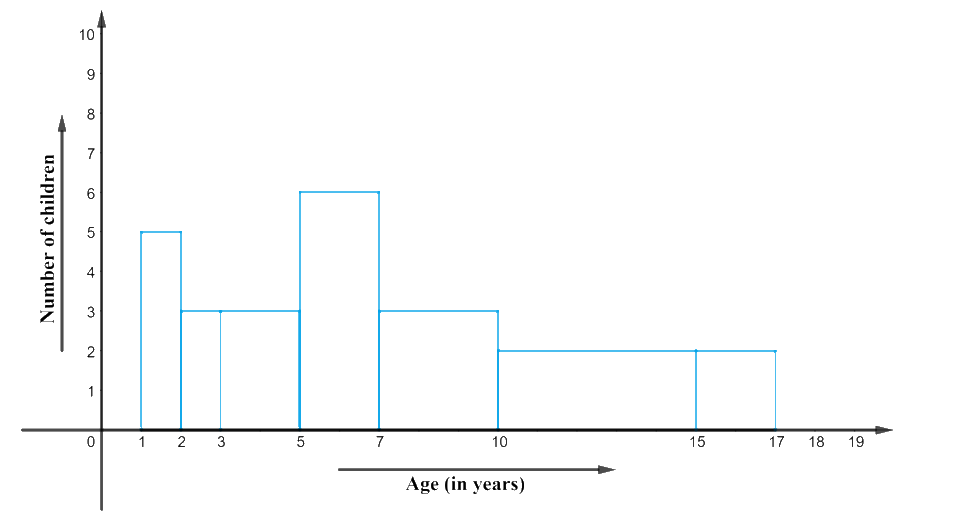## Chapter 14 Ex.14.3 Question 9

Surnames were randomly picked up from a local telephone directory and a frequency distribution of the number of letters in the English alphabet in the surnames was found as follows:

 Number of letters Number of surnames $$1 – 4$$ $$6$$ $$4 – 6$$ $$30$$ $$6 – 8$$ $$44$$ $$8 – 12$$ $$16$$ $$12 – 20$$ $$4$$

(i) Draw a histogram to depict the given information.

(ii) Write the class interval in which the maximum number of surnames lie.

### Solution

What is known?

Distribution of the number of letters in the English alphabet in the surnames.

What is Unknown?

(i) A Histogram to depict the given data.

(ii) The class interval in which the maximum number of surnames lies.

Reasoning:

(i) It can be observed from the given data that it has class intervals of varying width.

(ii) The proportion of number of surnames per $$2$$ letter interval (class interval of min class size for reference) can be made.

 Number of letters Number of surnames Width of the class Length of rectangle $$1 – 4$$ $$6$$ $$3$$ \begin{align}\frac{6 \times 2}{3}=4\end{align} $$4 – 6$$ $$30$$ $$2$$ \begin{align}\frac{30 \times 2}{2}=30\end{align} $$6 – 8$$ $$44$$ $$2$$ \begin{align}\frac{44 \times 2}{2}=44\end{align} $$8 – 12$$ $$16$$ $$4$$ \begin{align}\frac{16 \times 2}{4}=8\end{align} $$12 – 20$$ $$4$$ $$8$$ \begin{align}\frac{4 \times 2}{8}=1\end{align}

Steps:

The proportion of number of surnames per $$2$$ letter interval (class interval of min class size for reference) can be made as follows:

We will take the number of letters on $$x$$-axis and proportion of the number of surnames per $$2$$ letter interval on $$y$$-axis. And choose an appropriate scale of $$1 \,\rm unit = 4$$ surnames for $$y$$-axis.

The histogram can be constructed as follows: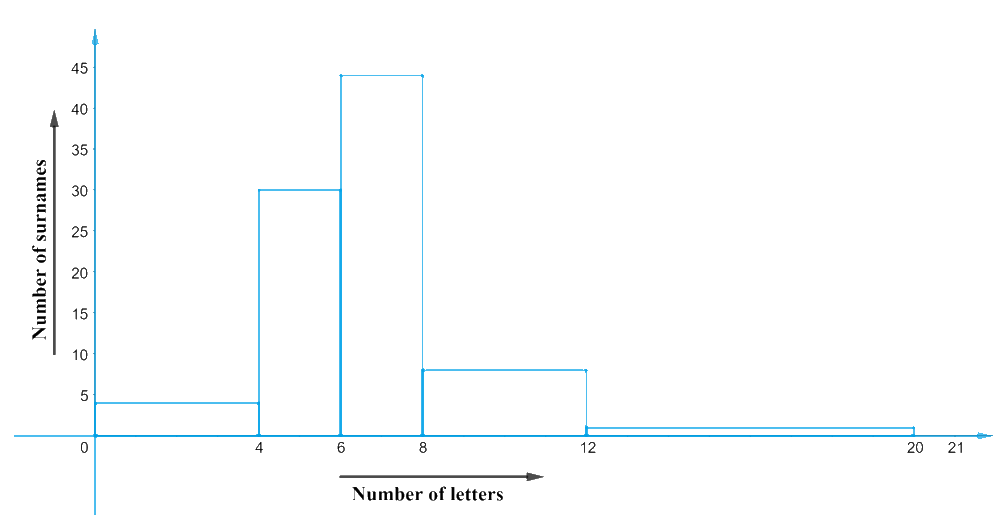The class interval in which the maximum number of surnames lies in the interval $$6 – 8$$ and has surnames in it.

Instant doubt clearing with Cuemath Advanced Math Program